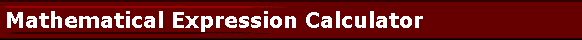# A Calculator Using Formula Parser for C++

To give an example of using bcParserCPP, the math parser for C++, library we have developed a C++ command line application that allows the user input an expression with x, y variables and it outputs the result.

If you wish to try out the application we are disucssing here, download demo Calculator.exe command line math expression calculator for Windows (It can be compiled for Linux and Mac OS as well as Windows).

Here is the screenshot in the Eclipse C++ IDE where you can see the source code and the console output: (Click on image to enlarge)

Here is the full source code for mathematical expression calculator:

 #include #define _DOUBLE #include "MathParser.h" using namespace std; //define a Math Parser that works with char strings for //variable and function names, and double values. typedef CMathParser MathParser; int main(char **args){                 try{                 MathParser p;                 string Expression;                 cout << "Input an expression such as x+sin(y) and press ENTER." << endl;                 cin >> Expression;                 //cout << Expression << endl;                 cout << endl;                                 p.SetExpression(Expression);                                 if(p.IsVariableUsed("X")){                         cout << "Input X value and press ENTER." << endl;                         double x;                         cin >> x;                         cout << endl;                         p.SetX(x);                 }                                 if(p.IsVariableUsed("Y")){                         cout << "Input Y value and press ENTER." << endl;                         double y;                         cin >> y;                         cout << endl;                         p.SetY(y);                 }                 cout << "-------------------------------------------------------" << endl;                 cout << "Result of " << p.GetExpression() << " is: "<< p.GetValue() << endl;                 cout << "-------------------------------------------------------" << endl;                 cout << "Done.";                         }catch(MathParser::ParserException &ex ){                 cout << ex.GetMessage() << endl;                 cout << "Invalid portion of expression is <" << ex.GetInvalidPortionOfExpression() << ">." << endl;         }catch(...){                 cout << "Unexpected error in math parser." << endl;         } }

The application expects you to input an expression such as x+y/2 on the console and hit enter. It takes that expression and checks whether variable X was used in it. If x was used, then it asks you to input X as a double value. Then does the same for Y. Once all information is collected, it outputs the value of the expression.

This Calculator application is shipped as a sample with along with the bcParserCPP deliverables.

webmaster@gobestcode.com